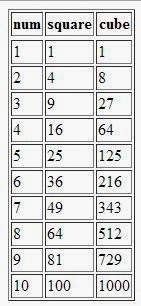Javascript program to calculate the squares and cubes of the numbers from 0 to 10 and display values in an HTML table - Anna University Internal marks 2018
This is one of the simple java script program to calculate the squares and cubes of the numbers from 0 to 10 and display values in an HTML table.JavaScript Tutorial

• Copy the above given coding and save it as anyname.html
• Open the saved file in any web browser.
• You will get an output like shown below.
Output:Javascript in Table Output

#### 1 comment:

1.pls give a solution for the same using external css for table